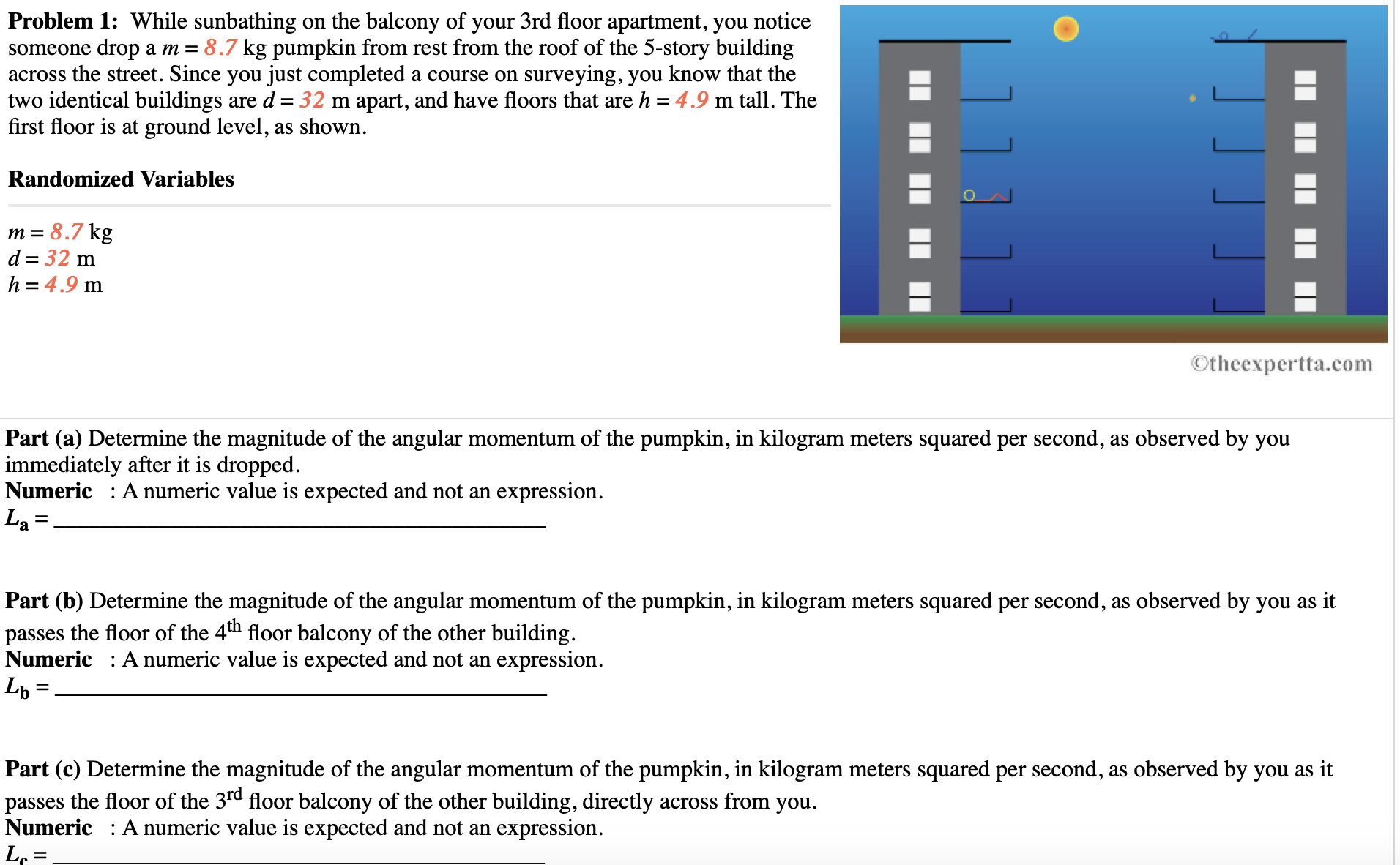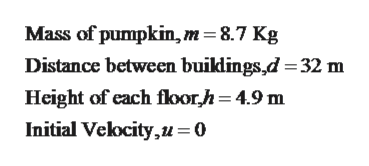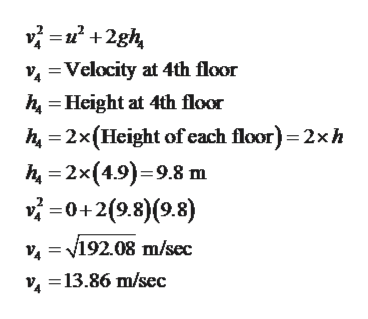# Problem 1: While sunbathing on the balcony of your 3rd floor apartment, you noticesomeone drop am = 8.7 kg pumpkin from rest from the roof of the 5-story buildingacross the street. Since you just completed a course on surveying, you know that thetwo identical buildings are d = 32 m apart, and have floors that are h = 4.9 m tall. Thefirst floor is at ground level, as shown.Randomized Variablesm 8.7 kgd 32 mh 4.9 mOtheexpertta.comPart (a) Determine the magnitude of the angular momentum of the pumpkin, in kilogram meters squared per second, as observed by youimmediately after it is droppedNumeric A numeric value is expected and not an expression.La:Part (b) Determine the magnitude of the angular momentum of the pumpkin, in kilogram meters squared per second, as observed by you as itpasses the floor of the 4th floor balcony of the other buildingNumeric : A numeric value is expected and not an expression.=Part (c) Determine the magnitude of the angular momentum of the pumpkin, in kilogram meters squared per second, as observed by you as itpasses the floor of the 3rd floor balcony of the other building, directly across from youNumeric A numeric value is expected and not an expression.Lo

Question
439 views
 Problem 1:  While sunbathing on the balcony of your 3rd floor apartment, you notice someone drop a m = 8.7 kg pumpkin from rest from the roof of the 5-story building across the street. Since you just completed a course on surveying, you know that the two identical buildings are d = 32 m apart, and have floors that are h = 4.9 m tall. The first floor is at ground level, as shown. Randomized Variablesm = 8.7 kgd = 32 mh = 4.9 m

 Part (a) Determine the magnitude of the angular momentum of the pumpkin, in kilogram meters squared per second, as observed by you immediately after it is dropped.Numeric   : A numeric value is expected and not an expression.La = __________________________________________Part (b) Determine the magnitude of the angular momentum of the pumpkin, in kilogram meters squared per second, as observed by you as it passes the floor of the 4th floor balcony of the other building.Numeric   : A numeric value is expected and not an expression.Lb = __________________________________________Part (c) Determine the magnitude of the angular momentum of the pumpkin, in kilogram meters squared per second, as observed by you as it passes the floor of the 3rd floor balcony of the other building, directly across from you.Numeric   : A numeric value is expected and not an expression.Lc = __________________________________________Part (d) Determine the magnitude of the angular momentum of the pumpkin, in kilogram meters squared per second, as observed by you as it passes the floor of the 2ndnd floor balcony of the other building.Numeric   : A numeric value is expected and not an expression.Ld = __________________________________________Part (e) Determine the magnitude of the angular momentum of the pumpkin, in kilogram meters squared per second, as observed by you immediately before it hits the ground, at the bottom of the first floor.Numeric   : A numeric value is expected and not an expression.Le = __________________________________________help_outlineImage TranscriptioncloseProblem 1: While sunbathing on the balcony of your 3rd floor apartment, you notice someone drop am = 8.7 kg pumpkin from rest from the roof of the 5-story building across the street. Since you just completed a course on surveying, you know that the two identical buildings are d = 32 m apart, and have floors that are h = 4.9 m tall. The first floor is at ground level, as shown. Randomized Variables m 8.7 kg d 32 m h 4.9 m Otheexpertta.com Part (a) Determine the magnitude of the angular momentum of the pumpkin, in kilogram meters squared per second, as observed by you immediately after it is dropped Numeric A numeric value is expected and not an expression. La : Part (b) Determine the magnitude of the angular momentum of the pumpkin, in kilogram meters squared per second, as observed by you as it passes the floor of the 4th floor balcony of the other building Numeric : A numeric value is expected and not an expression. = Part (c) Determine the magnitude of the angular momentum of the pumpkin, in kilogram meters squared per second, as observed by you as it passes the floor of the 3rd floor balcony of the other building, directly across from you Numeric A numeric value is expected and not an expression. Lo fullscreen
check_circle

Step 1

Given,help_outlineImage TranscriptioncloseMass of pumpkin, m= 8.7 Kg Distance between buikdings,d =32 m Height of each floorh=4.9 m Initial Velocity,u= 0 fullscreen
Step 2

Part (a):

Velocity of pumpkin just immediately after it is being dropped will be zero. So angular momentum just after it is being released from roof of other building will be zero.

Step 3

Part (b): Velocity of pumpkin at...help_outlineImage Transcriptionclosevi22gh Velocity at 4th floor h Height at 4th floor h 2x(Height of each floor) 2xh h 2x(4.9)9.8 m v0+2(9.8)(9.8) v = V192.08 m/sec =13.86 m/sec fullscreen

### Want to see the full answer?

See Solution

#### Want to see this answer and more?

Solutions are written by subject experts who are available 24/7. Questions are typically answered within 1 hour.*

See Solution
*Response times may vary by subject and question.
Tagged in

### Kinematics